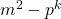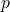# On the quantity m2 − pk where pkm2 is an odd perfect number

Jose Arnaldo Bebita Dris and Immanuel Tobias San Diego
Notes on Number Theory and Discrete Mathematics
Print ISSN 1310–5132, Online ISSN 2367–8275
Volume 26, 2020, Number 4, Pages 33—38
DOI: 10.7546/nntdm.2020.26.4.33-38

## Details

### Authors and affiliations

Jose Arnaldo Bebita DrisDe La Salle University, Manila, Philippines 1004

Immanuel Tobias San DiegoDepartment of Mathematics and Physical Sciences
Trinity University of Asia, Quezon City, Philippines 1102

### Abstract

We prove thatis not a square, ifis an odd perfect number with special prime, under the hypothesis thatis a square. We are also able to prove the same assertion without this hypothesis. We also show that this hypothesis is incompatible with the set of assumptionsWe end by stating some conjectures.

### Keywords

• Sum of divisors
• Sum of aliquot divisors
• Deficiency
• Odd perfect number
• Special prime

• 11A05
• 11A25

### References

1. Beasley, B. D. (2013). Euler and the ongoing search for odd perfect numbers, Proc. of the ACMS 19-th Biennial Conference, 29 May–1 June, 2013, Bethel University, St. Paul, MN, pp. 21–31. Available online: http://godandmath.files.wordpress.com/2013/07/acms-2013-proceedings.pdf.
2. Broughan, K. A., Delbourgo, D., & Zhou, Q. (2013). Improving the Chen and Chen result for odd perfect numbers, Integers, 13, Article #A39.
3. Brown, P. A. (2016). A partial proof of a conjecture of Dris. Preprint, https://arxiv.org/abs/1602.01591.
4. Chen, F.-J., & Chen, Y.-G. (2014). On the index of an odd perfect number, Colloquium Mathematicum, 136, 41–49.
5. Chen, S.-C., & Luo, H. (2013). Odd multiperfect numbers, Bulletin of the Australian Mathematical Society, 88 (1), 56–63.
6. Dris, J. A. B. (2017). On a curious biconditional involving the divisors of odd perfect numbers, Notes on Number Theory and Discrete Mathematics, 23 (4), 1–13.
7. Dris, J. A. B. (2017). Conditions equivalent to the Descartes–Frenicle–Sorli Conjecture on odd perfect numbers, Notes on Number Theory and Discrete Mathematics, 23 (2), 12–20.
8. Dris, J. A. B. (2012). The abundancy index of divisors of odd perfect numbers, Journal of Integer Sequences, 15 (4), Article 12.4.4.
9. Dris, J. A. B., & San Diego, I. T. (2020). Some modular considerations regarding odd perfect numbers, Notes on Number Theory and Discrete Mathematics, 26(2), 27–33.
10. Dris, J. A. B., & MathStackExchange user FredH (2019). If n = pkm2 is an odd perfect number with special prime p, then m2pk is not a square. Available online: https://math.stackexchange.com/questions/3121498.
11. Dris, J. A. B., & Tejada, D.-J. U. (2018). Revisiting some old results on odd perfect numbers, Notes on Number Theory and Discrete Mathematics, 24 (4), 18–25.
12. Dris, J. A. B., & Tejada, D.-J. U. (2018). Conditions equivalent to the Descartes–Frenicle–Sorli Conjecture on odd perfect numbers – Part II, Notes on Number Theory and Discrete Mathematics, 24 (3), 62–67.
13. Sloane, N. J. A., OEIS sequence A033879 – Deficiency of n, or 2nσ(n), Available online: https://oeis.org/A033879.
14. Sloane, N. J. A., & Guy, R. K., OEIS sequence A001065 – Sum of proper divisors (or aliquot parts) of n: sum of divisors of n that are less than n, Available online: https://oeis.org/A001065.
15. Sorli, R. M. (2003). Algorithms in the Study of Multiperfect and Odd Perfect Numbers, Ph.D. Thesis, University of Technology, Sydney, Available online: http://hdl.handle.net/10453/20034.
16. Starni, P. (2018). On Dris conjecture about odd perfect numbers, Notes on Number Theory and Discrete Mathematics, 24 (1), 5–9.
17. Wikipedia contributors. (2020, July 15). Perfect number. In Wikipedia, The Free Encyclopedia. Retrieved from: https://en.wikipedia.org/w/index.php?title=
Perfect_number&oldid=967867153
.

## Cite this paper

Dris, J. A. B., & San Diego, I. T. (2020). On the quantity m2pk where pkm2 is an odd perfect number, 26(4), 33-38, doi: 10.7546/nntdm.2020.26.4.33-38.